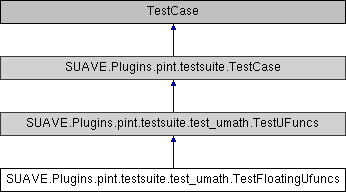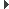SUAVE  2.5.2 An Aerospace Vehicle Environment for Designing Future AircraftSUAVE.Plugins.pint.testsuite.test_umath.TestFloatingUfuncs Class Reference
Inheritance diagram for SUAVE.Plugins.pint.testsuite.test_umath.TestFloatingUfuncs:## Public Member Functions

def test_isreal (self)

def test_iscomplex (self)

def test_isfinite (self)

def test_isinf (self)

def test_isnan (self)

def test_signbit (self)

def test_copysign (self)

def test_nextafter (self)

def test_modf (self)

def test_ldexp (self)

def test_frexp (self)

def test_fmod (self)

def test_floor (self)

def test_ceil (self)

def test_trunc (self)Public Member Functions inherited from SUAVE.Plugins.pint.testsuite.test_umath.TestUFuncs
def qless (self)

def qs (self)

def q1 (self)

def q2 (self)

def qm (self)

def qi (self)

def assertEqual (self, first, second, msg=None)

def assertRaisesMsg (self, msg, ExcType, func, *args, **kwargs)Public Member Functions inherited from SUAVE.Plugins.pint.testsuite.TestCase
def setUpClass (cls)

def assertSequenceEqual (self, seq1, seq2, msg=None, seq_type=None)

def assertAlmostEqual (self, first, second, places=None, msg=None, delta=None)

def assertAlmostEqualRelError (self, first, second, rel, msg=None)Public Attributes inherited from SUAVE.Plugins.pint.testsuite.TestCase
ureg

Q_Static Public Attributes inherited from SUAVE.Plugins.pint.testsuite.test_umath.TestUFuncs
bool FORCE_NDARRAY = True

## Detailed Description

```Universal functions (ufunc) > Floating functions

http://docs.scipy.org/doc/numpy/reference/ufuncs.html#floating-functions

isreal(x)   Returns a bool array, where True if input element is real.
iscomplex(x)    Returns a bool array, where True if input element is complex.
isfinite(x[, out])  Test element-wise for finite-ness (not infinity or not Not a Number).
isinf(x[, out])     Test element-wise for positive or negative infinity.
isnan(x[, out])     Test element-wise for Not a Number (NaN), return result as a bool array.
signbit(x[, out])   Returns element-wise True where signbit is set (less than zero).
copysign(x1, x2[, out])     Change the sign of x1 to that of x2, element-wise.
nextafter(x1, x2[, out])    Return the next representable floating-point value after x1 in the direction of x2 element-wise.
modf(x[, out1, out2])   Return the fractional and integral parts of an array, element-wise.
ldexp(x1, x2[, out])    Compute y = x1 * 2**x2.
frexp(x[, out1, out2])  Split the number, x, into a normalized fraction (y1) and exponent (y2)
fmod(x1, x2[, out])     Return the element-wise remainder of division.
floor(x[, out])     Return the floor of the input, element-wise.
ceil(x[, out])  Return the ceiling of the input, element-wise.
trunc(x[, out])     Return the truncated value of the input, element-wise.
```

The documentation for this class was generated from the following file:
• /Users/emiliobotero/Dropbox/SUAVE/SUAVE/trunk/SUAVE/Plugins/pint/testsuite/test_umath.py